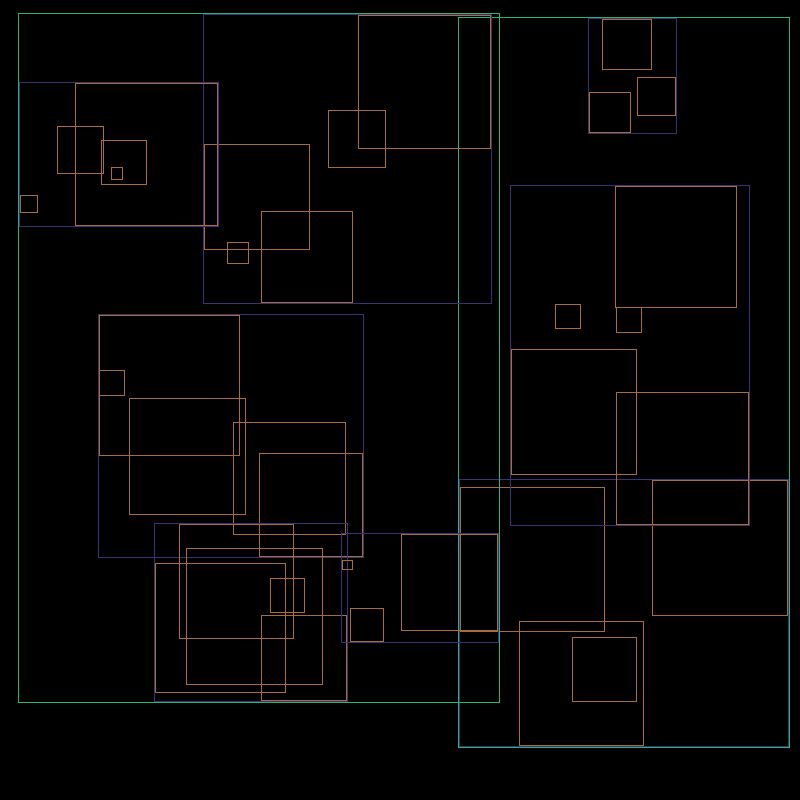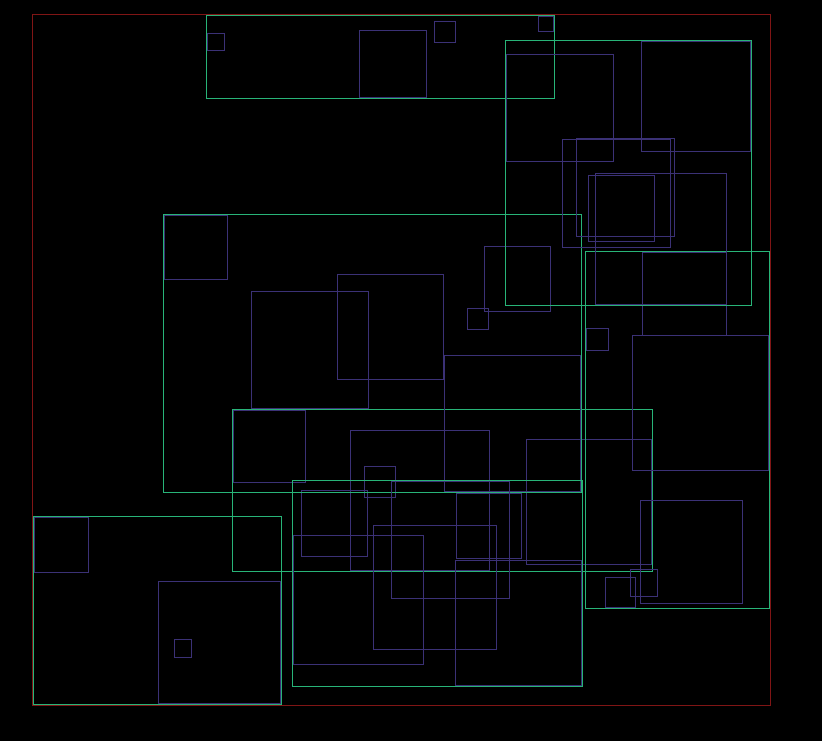# spaceindex

spaceindex is a tool for building r-trees.## Installation

Add this to your `Cargo.toml`:

``````[dependencies]
spaceindex = "0.3"
``````

## Basic Usage

To create a new `RTree`, use:

```use spaceindex::rtree::RTree;

// Creates a 2-dimensional RTree
let mut rtree : RTree<()> = RTree::new(2);

// This region is the rectangle whose lower-left corner is at (0,0) and whose upper-right corner is at (2, 2)
rtree.insert(((0.0, 0.0), (2.0, 2.0)), ()).expect("failed to insert");

// This region goes from (1, 0) to (3, 3).
rtree.insert(((1.0, 0.0), (3.0, 3.0)), ()).expect("failed to insert");

// Both rectangles contain the point (1, 1)
assert_eq!(rtree.point_lookup((1.0, 1.0)).len(), 2);

// No rectangle should contain the point (-1, 0)
assert!(rtree.point_lookup((-1.0, 0.0)).is_empty());```

## Python module

Also included is `pyspaceindex`, a Python module exposing a simple interface for working with two dimensional RTree's.

### Build instructions

To build `pyspaceindex`:

• Install the excellent maturin package from pypi.
• Navigate to the `pyspaceindex` directory in this repository, then run `maturin build` to build a copy of the wheel. To install the module in your current virtualenv instead, run `maturin develop` instead.

### Example usage

``````import pyspaceindex as psi

# Make an RTree instance
tree = psi.RTree()

# A region is described by a tuple (min_x, min_y, max_x, max_y).
tree.insert((0, 0, 3, 3), 12)

# A tree can contain data, as well.
tree.insert((-1, -1, 2, 2), 99)

# Query the tree for whether it contains a point
assert sorted(tree.query(0.5, 1.0)) == [12, 99]
``````